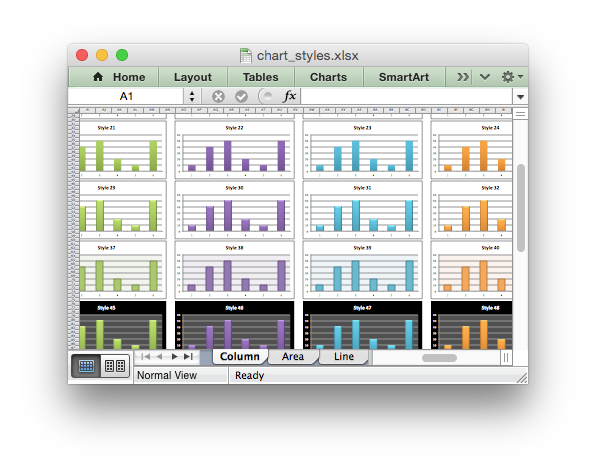libxlsxwriter
chart_styles.c

An example showing all 48 default chart styles available in Excel 2007 using the chart `chart_set_style()` method./*
* An example showing all 48 default chart styles available in Excel 2007
* using the libxlsxwriter library. Note, these styles are not the same as the
* styles available in Excel 2013.
*
* Copyright 2014-2021, John McNamara, jmcnamara@cpan.org
*
*/
#include "xlsxwriter.h"
int main() {
char *chart_names[] = {"Column", "Area", "Line", "Pie"};
char chart_title = {0};
int row_num, col_num, chart_num, style_num;
lxw_worksheet *worksheet;
lxw_chart *chart;
lxw_workbook *workbook = workbook_new("chart_styles.xlsx");
for (chart_num = 0; chart_num < 4; chart_num++) {
/* Add a worksheet for each chart type. */
worksheet_set_zoom(worksheet, 30);
/* Create 48 charts, each with a different style. */
style_num = 1;
for (row_num = 0; row_num < 90; row_num += 15) {
for (col_num = 0; col_num < 64; col_num += 8) {
snprintf(chart_title, 32, "Style %d", style_num);
chart_title_set_name(chart, chart_title);
chart_set_style(chart, style_num);
worksheet_insert_chart(worksheet, row_num, col_num, chart);
style_num++;
}
}
}
/* Create a worksheet with data for the charts. */
worksheet_write_number(worksheet, 0, 0, 10, NULL);
worksheet_write_number(worksheet, 1, 0, 40, NULL);
worksheet_write_number(worksheet, 2, 0, 50, NULL);
worksheet_write_number(worksheet, 3, 0, 20, NULL);
worksheet_write_number(worksheet, 4, 0, 10, NULL);
worksheet_write_number(worksheet, 5, 0, 50, NULL);
return workbook_close(workbook);
}
chart_set_style
void chart_set_style(lxw_chart *chart, uint8_t style_id)
Set the chart style type.
workbook_close
lxw_error workbook_close(lxw_workbook *workbook)
Close the Workbook object and write the XLSX file.
LXW_CHART_AREA
@ LXW_CHART_AREA
Definition: chart.h:95
LXW_CHART_PIE
@ LXW_CHART_PIE
Definition: chart.h:134
lxw_chart_series * chart_add_series(lxw_chart *chart, const char *categories, const char *values)
Add a data series to a chart.
workbook_new
lxw_workbook * workbook_new(const char *filename)
Create a new workbook object.
worksheet_set_zoom
void worksheet_set_zoom(lxw_worksheet *worksheet, uint16_t scale)
Set the worksheet zoom factor.
LXW_CHART_LINE
@ LXW_CHART_LINE
Definition: chart.h:125
lxw_worksheet
Struct to represent an Excel worksheet.
Definition: worksheet.h:2107
lxw_chart
Struct to represent an Excel chart.
Definition: chart.h:1090
worksheet_insert_chart
lxw_error worksheet_insert_chart(lxw_worksheet *worksheet, lxw_row_t row, lxw_col_t col, lxw_chart *chart)
Insert a chart object into a worksheet.
lxw_workbook
Struct to represent an Excel workbook.
Definition: workbook.h:292
chart_title_set_name
void chart_title_set_name(lxw_chart *chart, const char *name)
Set the title of the chart.
worksheet_write_number
lxw_error worksheet_write_number(lxw_worksheet *worksheet, lxw_row_t row, lxw_col_t col, double number, lxw_format *format)
Write a number to a worksheet cell.